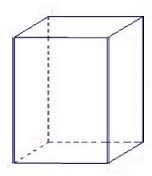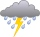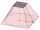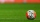# Prism 4 sides

The prism has a square base with a side length of 3 cm. The diagonal of the sidewall of the prism/BG/is 5 cm. Calculate the surface of this prism in cm square and the volume in liters

Result

S =  66 cm2
V =  0.036 l

#### Solution:

$a=3 \ \text{cm} \ \\ u=5 \ \text{cm} \ \\ \ \\ c=\sqrt{ u^2-a^2 }=\sqrt{ 5^2-3^2 }=4 \ \text{cm} \ \\ \ \\ S_{1}=a^2=3^2=9 \ \text{cm}^2 \ \\ \ \\ S_{2}=4 \cdot \ a \cdot \ c=4 \cdot \ 3 \cdot \ 4=48 \ \text{cm}^2 \ \\ \ \\ S=2 \cdot \ S_{1} + S_{2}=2 \cdot \ 9 + 48=66 \ \text{cm}^2$
$V_{1}=S_{1} \cdot \ c=9 \cdot \ 4=36 \ \text{cm}^3 \ \\ V=V_{1} \rightarrow l=V_{1} / 1000 \ l=36 / 1000 \ l=0.036 \ l=\dfrac{ 9 }{ 250 }=0.036 \ \text{l}$Our examples were largely sent or created by pupils and students themselves. Therefore, we would be pleased if you could send us any errors you found, spelling mistakes, or rephasing the example. Thank you!

Leave us a comment of this math problem and its solution (i.e. if it is still somewhat unclear...):Be the first to comment!Tips to related online calculators
Do you know the volume and unit volume, and want to convert volume units?
Pythagorean theorem is the base for the right triangle calculator.

## Next similar math problems:

1. Pentagonal prismThe regular pentagonal prism is 10 cm high. The radius of the circle of the described base is 8 cm. Calculate the volume and surface area of the prism.
2. EmbankmentThe railway embankment 300 m long has a cross section of an isosceles trapezoid with bases of 14 m and 8 m. The trapezoidal arms are 5 m long. Calculate how much m3 of soil is in the embankment?
3. Water tank300hl of water was filled into the tank 12 m long and 6 m wide. How high does it reach?
4. RainGarden shape of a rectangle measuring 15 m and 20 m rained water up to 3 mm. How many liters of water rained on the garden?
5. Runcated pyramid teapotThe 35 cm high teapot has the shape of a truncated pyramid with the length of the edge of the lower square base a=50 cm and with the edges of the rectangular base b: 20 cm and c: 30 cm. How many liters of water will fit in the teapot?
6. Diameter = heightThe surface of the cylinder, the height of which is equal to the diameter of the base, is 4239 cm square. Calculate the cylinder volume.
7. The conicalThe conical candle has a base diameter of 20 cm and a side of 30 cm. How much dm ^ 3 of wax was needed to make it?
8. Oak trunkCalculate in tonnes the approximate weight of a cylindrical oak trunk with a diameter of 66 cm and a length of 4 m, knowing that the density of the wood was 800 kg/m³.
9. The copper wireThe copper wire bundle with a diameter of 2.8mm has a weight of 5kg. How many meters of wire is bundled if 1m3 of copper weighs 8930kg?
10. Volume and surface areaFind the volume and surface of a wooden block with dimensions: a = 8 cm, b = 10 cm, c = 16 cm.
11. What is bigger?Which ball has a larger volume: a football with a circumference of 66 cm or a volleyball with a diameter of 20 cm?
12. CannonballsOf the three cannonballs with a diameter of 16 cm, which landed in the castle courtyard during the battle, the castle blacksmith cast balls with a diameter of 10 cm, which fit into the cannons placed on the walls. How many cannonballs did the blacksmith c
13. The EarthThe Earth's surface is 510,000,000 km2. Calculates the radius, equator length, and volume of the Earth, assuming the Earth has the shape of a sphere.
14. Tunnel boringHow much material did they dig when cutting the 400m long tunnel? The content of the circular segment, which is the cross section of the tunnel is 62m2.
15. Cone from cubeThe largest possible cone was turned from a 20 cm high wooden cube. Calculate its weight if you know that the density of wood was 850 kg/m3
16. Water mixingThere are 5 liters of water at 75°C in the pot, how much water at 10°C do we have to add to the resulting temperature of 55°C?
17. A bucketA bucket has 4 liters of water in it when it is 2/5 full. How much can it hold?